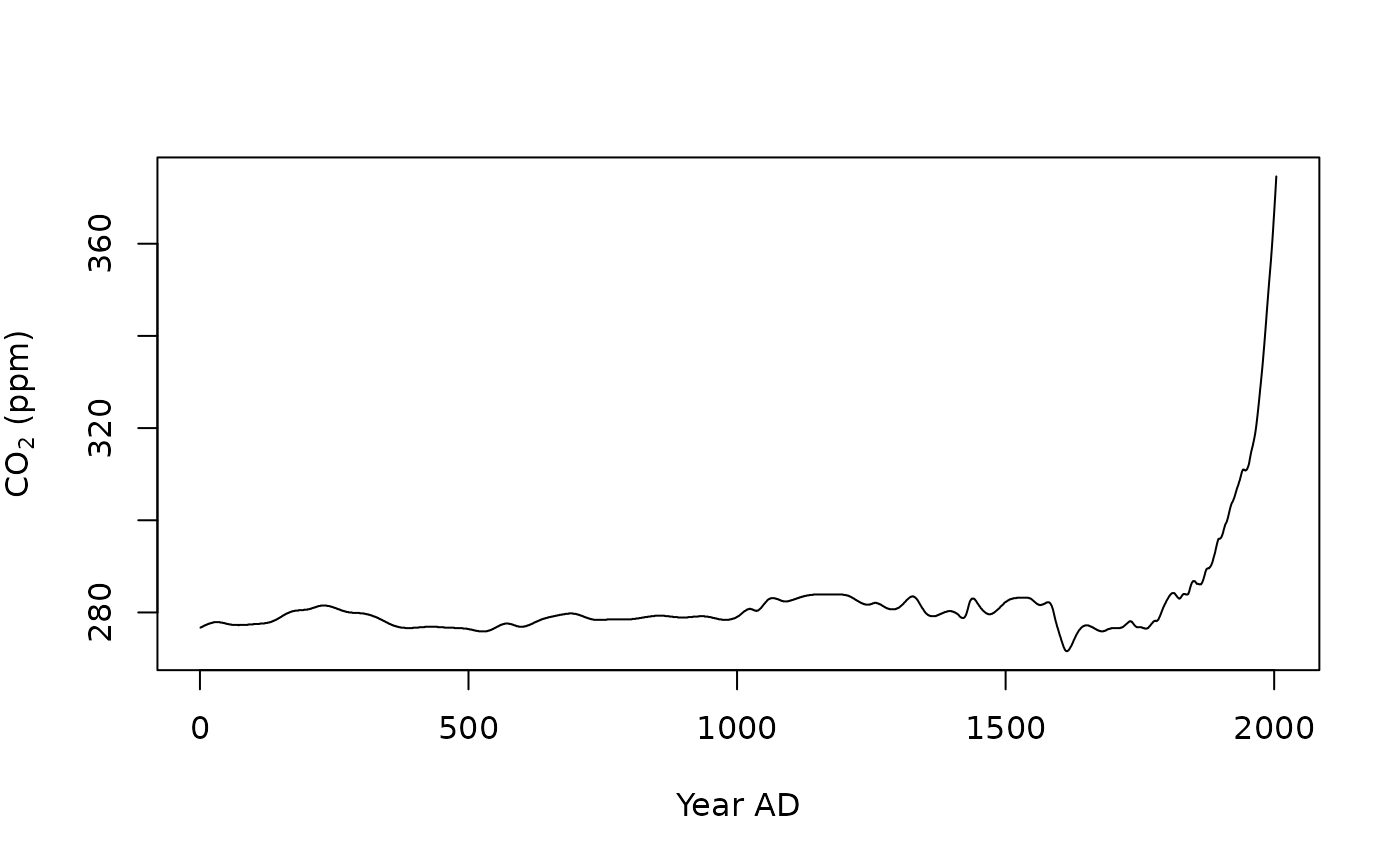Law Dome Ice Core 2000-year $$CH_4$$, $$CO_2$$ and $$N_{2}O$$ data.

law2006

Format

A data frame with 2004 observations and 8 variables:

year

NOAA04

NOAA04 $$CH_4$$ scale.

CH4_spl

$$CH_4$$ spline (ppb).

CH4_grw

$$CH_4$$ growth Rate (ppb/yr).

CO2_spl

$$CO_2$$ spline (ppb).

CO2_grw

$$CO_2$$ growth Rate (ppb/yr).

N2O_spl

$$N_{2}O$$ spline (ppb).

N2O_grw

$$N_{2}O$$ growth Rate (ppb/yr).

MacFarling Meure, C., Etheridge, D., Trudinger, C., Steele, P., Langenfelds, R., van Ommen, T., Smith, A. and Elkins, J. (2006). The Law Dome CO2, CH4 and N2O Ice Core Records Extended to 2000 years BP. Geophysical Research Letters, 33(14), L14810. doi: 10.1029/2006GL026152 .

Examples

plot(
x = law2006$year, y = law2006$CO2_spl,
type = "l",